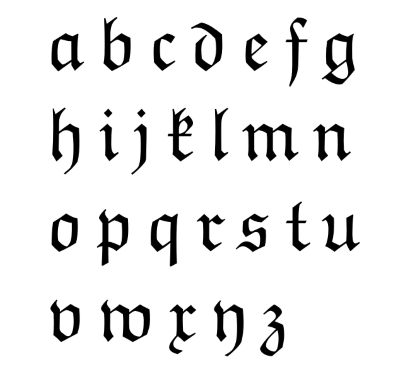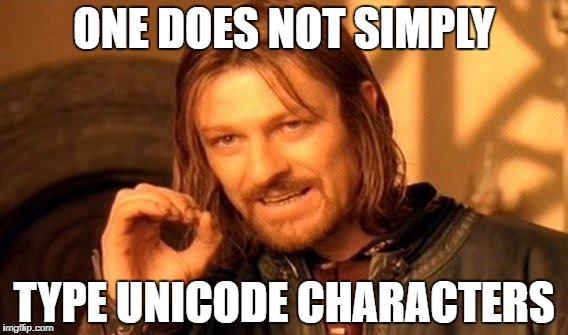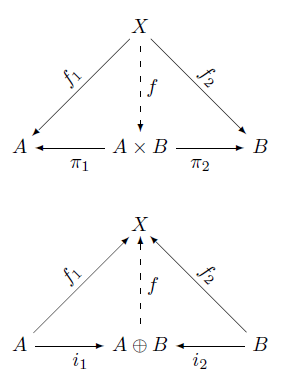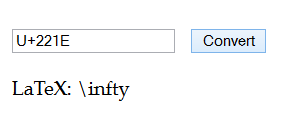# Fraktur symbols in mathematics

When mathematicians run out of symbols, they turn to other alphabets. Most math symbols are Latin or Greek letters, but occasionally you’ll run into Russian or Hebrew letters.

Sometimes math uses a new font rather than a new alphabet, such as Fraktur. This is common in Lie groups when you want to associate related symbols to a Lie group and its Lie algebra. By convention a Lie group is denoted by an ordinary Latin letter and its associated Lie algebra is denoted by the same letter in Fraktur font.## LaTeX

To produce Fraktur letters in LaTeX, load the amssymb package and use the command \mathfrak{}.

Symbols such as \mathfrak{A} are math symbols and can only be used in math mode. They are not intended to be a substitute for setting text in Fraktur font. This is consistent with the semantic distinction in Unicode described below.

## Unicode

The Unicode standard tries to distinguish the appearance of a symbol from its semantics, though there are compromises. For example, the Greek letter Ω has Unicode code point U+03A9 but the symbol Ω for electrical resistance in Ohms is U+2621 even though they are rendered the same .

The letters a through z, rendered in Fraktur font and used as mathematical symbols, have Unicode values U+1D51E through U+1D537. These values are in the “Supplementary Multilingual Plane” and do not commonly have font support .

The corresponding letters A through Z are encoded as U+1D504 through U+1D51C, though interestingly a few letters are missing. The code point U+1D506, which you’d expect to be Fraktur C, is reserved. The spots corresponding to H, I, and R are also reserved. Presumably these are reserved because they are not commonly used as mathematical symbols. However, the corresponding bold versions U+1D56C through U+ID585 have no such gaps .

## Footnotes

 At least they usually are. A font designer could choose provide different glyphs for the two symbols. I used the same character for both because some I thought some readers might not see the Ohm symbol properly rendered.

 If you have the necessary fonts installed you should see the alphabet in Fraktur below:
𝔞 𝔟 𝔠 𝔡 𝔢 𝔣 𝔤 𝔥 𝔦 𝔧 𝔨 𝔩 𝔪 𝔫 𝔬 𝔭 𝔮 𝔯 𝔰 𝔱 𝔲 𝔳 𝔴 𝔵 𝔶 𝔷

I can see these symbols from my desktop and from my iPhone, but not from my Android tablet. Same with the symbols below.

 Here are the bold upper case and lower case Fraktur letters in Unicode:
𝕬 𝕭 𝕮 𝕯 𝕰 𝕱 𝕲 𝕳 𝕴 𝕵 𝕶 𝕷 𝕸 𝕹 𝕺 𝕻 𝕼 𝕽 𝕾 𝕿 𝖀 𝖁 𝖂 𝖃 𝖄 𝖅
𝖆 𝖇 𝖈 𝖉 𝖊 𝖋 𝖌 𝖍 𝖎 𝖏 𝖐 𝖑 𝖒 𝖓 𝖔 𝖕 𝖖 𝖗 𝖘 𝖙 𝖚 𝖛 𝖜 𝖝 𝖞 𝖟

# Putting a brace under something in LaTeX

Here’s a useful LaTeX command that I learned about recently: \underbrace.

It does what it sounds like it does. It puts a brace under its argument.

I used this a few days ago in the post on the new prime record when I wanted to show that the record prime is written in hexadecimal as a 1 followed by a long string of Fs.The code that produced is

1\underbrace{\mbox{FFF \ldots FFF}}_\mbox{{\normalsize 9,308.229 F's}}


The sizing is a little confusing. Without \normalsize the text under the brace would be as large as the text above.

# Why don’t you simply use XeTeX?

From an FAQ post I wrote a few years ago:

This may seem like an odd question, but it’s actually one I get very often. On my TeXtip twitter account, I include tips on how to create non-English characters such as using \AA to produce Å. Every time someone will ask “Why not use XeTeX and just enter these characters?”

If you can “just enter” non-English characters, then you don’t need a tip. But a lot of people either don’t know how to do this or don’t have a convenient way to do so. Most English speakers only need to type foreign characters occasionally, and will find it easier, for example, to type \AA or \ss than to learn how to produce Å or ß from a keyboard. If you frequently need to enter Unicode characters, and know how to do so, then XeTeX is great.# Unicode / LaTeX page updated

Almost three years ago I put up a web page to let you go back and forth between Unicode code points and LaTeX commands. Here’s the page and here’s a blog post explaining it.

I’ve expanded the data the page uses by merging in data from the STIX Project. More queries should return successfully now.

# Haskell analog of Sweave and Pweave

Sweave and Pweave are programs that let you embed R and Python code respectively into LaTeX files. You can display the source code, the result of running the code, or both.

lhs2TeX is roughly the Haskell analog of Sweave and Pweave.  This post takes the sample code I wrote for Sweave and Pweave before and gives a lhs2TeX counterpart.

\documentclass{article}
%include polycode.fmt
%options ghci
\long\def\ignore#1{}
\begin{document}

Invisible code that sets the value of the variable $a$.

\ignore{
\begin{code}
a = 3.14
\end{code}
}

Visible code that sets $b$ and squares it.

(There doesn't seem to be a way to display the result of a block of code directly.
Seems you have to save the result and display it explicitly in an eval statement.)

\begin{code}
b = 3.15
c = b*b
\end{code}

$b^2$ = \eval{c}

Calling Haskell inline: $\sqrt{2} = \eval{sqrt 2}$

Recalling the variable $a$ set above: $a$ = \eval{a}.

\end{document}


If you save this code to a file foo.lhs, you can run

lhs2TeX -o foo.tex foo.lhs

to create a LaTeX file foo.tex which you could then compile with pdflatex.

One gotcha that I ran into is that your .lhs file must contain at least one code block, though the code block may be empty. You cannot just have code in \eval statements.

Unlike R and Python, the Haskell language itself has a notion of literate programming. Haskell specifies a format for literate comments. lhs2TeX is a popular tool for processing literate Haskell files but not the only one.

# Commutative diagrams in LaTeX

There are numerous packages for creating commutative diagrams in LaTeX. My favorite, based on my limited experience, is Paul Taylor’s package. Another popular package is tikz-cd.

To install Paul Taylor’s package on Windows, I created a directory called localtexmf, set the environment variable TEXINPUTS to its location, and copied diagrams.sty file in that directory.

Here are a couple examples, diagrams used in the definition of product and coproduct.And here’s the LaTeX to produce the diagrams.

\begin{diagram}
& & X & & \\
& \ldTo^{f_1} & \dDashto_f & \rdTo^{f_2} & \\
A & \lTo_{\pi_1} & A\times B & \rTo_{\pi_2} & B \\
\end{diagram}

\begin{diagram}
& & X & & \\
& \ruTo^{f_1} & \uDashto_f & \luTo^{f_2} & \\
A & \rTo_{i_1} & A\oplus B & \lTo_{i_2} & B \\
\end{diagram}



For much more information, see the package page.

RelatedApplied category theory

# Unicode to LaTeX

I’ve run across a couple websites that let you enter a LaTeX symbol and get back its Unicode value. But I didn’t find a site that does the reverse, going from Unicode to LaTeX, so I wrote my own.

Unicode / LaTeX Conversion

If you enter Unicode, it will return LaTeX. If you enter LaTeX, it will return Unicode. It interprets a string starting with “U+” as a Unicode code point, and a string starting with a backslash as a LaTeX command.For example, the screenshot above shows what happens if you enter U+221E and click “convert.” You could also enter infty and get back U+221E.

However, if you go from Unicode to LaTeX to Unicode, you won’t always end up where you started. There may be multiple Unicode values that map to a single LaTeX symbol. This is because Unicode is semantic and LaTeX is not. For example, Unicode distinguishes between the Greek letter Ω and the symbol Ω for ohms, the unit of electrical resistance, but LaTeX does not.

# Automatic delimiter sizes in LaTeX

I recently read a math book in which delimiters never adjusted to the size of their content or the level of nesting. This isn’t unusual in articles, but books usually pay more attention to typography.

Here’s a part of an equation from the book:Larger outer parentheses make the equation much easier to read, especially as part of a complex equation. It’s clear at a glance that the function φ-1 applies to the result of the integral.The first equation was typeset using

    \varphi^{-1} ( \int \varphi(f+g) \,d\mu )

The latter used left and right to tell LaTeX that the parentheses should grow to match the size of the content between them.

    \varphi^{-1} \left( \int \varphi(f+g) \,d\mu \right)

You can use \left and \right with more delimiters than just parentheses: braces, brackets, ceiling, floor, etc. And the left and right delimiters do not need to match. You could make a half-open interval, for example, with \left( on one side and \right] on the other.

For every \left delimiter there must be a corresponding \right delimiter. However, you can make one of the pair empty by using a period as its mate. For example, you could start an expression with \left[ and end it with \right. which would create a left bracket as tall as the tallest thing between that bracket and the corresponding \right. command. Note that \right. causes nothing to be displayed, not even a period.

The most common example of a delimiter with no mate may be a curly brace on the left with no matching brace on the right. In that case you’d need to open with \left\{. The backslash in front of the brace is necessary to tell LaTeX that you want a literal brace and that you’re not just using the brace for grouping.

# Basics of Sweave and Pweave

Sweave is a tool for embedding R code in a LaTeX file. Pweave is an analogous tool for Python. By putting your code in your document rather than the results of running your code somewhere else, results are automatically recomputed when inputs change. This is especially useful with graphs: rather than including an image into your document, you include the code to create the image.

To use either Sweave or Pweave, you create a LaTeX file and include source code inside. A code block begins with <<>>= and ends with @ on a line by itself. By default, code blocks appear in the LaTeX output. You can start a code block with <<echo=FALSE>>= to execute code without echoing its source. In Pweave you can also use <% and %> to mark a code block that executes but does not echo. You might want to do this at the top of a file, for example, for import statements.

Sweave echos code like the R command line, with > for the command prompt. Pweave does not display the Python >>> command line prompt by default, though it will if you use the option term=TRUE in the start of your code block.

In Sweave, you can use Sexpr to inline a little bit of R code. For example, $x = Sexpr{sqrt(2)}$ will produce x = 1.414…. You can also use Sexpr to reference variables defined in previous code blocks. The Pweave analog uses <%= and %>. The previous example would be $x = <%= sqrt(2) %>$.

You can include a figure in Sweave or Pweave by beginning a code block with <<fig=TRUE, echo=FALSE>>= or with echo=TRUE if you want to display the code that produces the figure. With Sweave you don’t need to do anything else with your file. With Pweave you need to add usepackage{graphicx} at the top.

To process an Sweave file foo.Rnw, run Sweave("foo.Rnw") from the R command prompt. To process a Pweave file foo.Pnw, run Pweave -f tex foo.Pnw from the shell. Either way you get a LaTeX file that you can then compile to a PDF.

Here are sample Sweave and Pweave files. First Sweave:

\documentclass{article}
\begin{document}

Invisible code that sets the value of the variable $a$.

<<<echo=FALSE>>=
a <- 3.14
@

Visible code that sets $b$ and squares it.

<<bear, echo=TRUE>>=
b <- 3.15
b*b
@

Calling R inline: $\sqrt{2} = Sexpr{sqrt(2)}$

Recalling the variable $a$ set above: $a = Sexpr{a}$.

Here's a figure:

<<fig=TRUE, echo=FALSE>>=
x <- seq(0, 6*pi, length=200)
plot(x, sin(x))
@

\end{document}

And now Pweave:

\documentclass{article}
\usepackage{graphicx}
\begin{document}

<%
import matplotlib.pyplot as plt
from numpy import pi, linspace, sqrt, sin
%>

Invisible code that sets the value of the variable $a$.

<<echo=FALSE>>=
a = 3.14
@

Visible code that sets $b$ and squares it.

<<term=True>>=
b = 3.15
print b*b
@

Calling Python inline: $\sqrt{2} = <%= sqrt(2) %>$

Recalling the variable $a$ set above: $a = <%= a %>$.

Here's a figure:

<<fig=True, echo=False>>=
x = linspace(0, 6*pi, 200)
plt.plot(x, sin(x))
plt.show()
@

\end{document}